## Saturday, 9 August 2014

### chapter 11 Worked Out Examples 2

 Example: 3
 Find the values of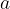$a$ and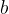$b$ if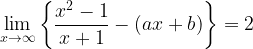$\mathop {\lim }\limits_{x \to \infty } \left\{ {\dfrac{{{x^2} - 1}}{{x + 1}} - \left( {ax + b} \right)} \right\} = 2$
 Solution: 3
The limit can be rearranged as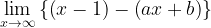$\mathop {\lim }\limits_{x \to \infty } \left\{ {\left( {x - 1} \right) - \left( {ax + b} \right)} \right\}$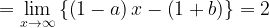$= \mathop {\lim }\limits_{x \to \infty } \left\{ {\left( {1 - a} \right)x - \left( {1 + b} \right)} \right\} = 2$
Since the limit is finite, the coefficient of$a$ has to be necessarily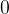$0$.
Therefore,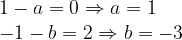$\begin{array}{l} 1 - a = 0 \Rightarrow a = 1\\ - 1 - b = 2 \Rightarrow b = - 3 \end{array}$
 Example: 4
 Evaluate$\mathop {\lim }\limits_{n \to \infty } \dfrac{{\left[ x \right] + \left[ {{2^2}x} \right] + \left[ {{3^2}x} \right] + \ldots + \left[ {{n^2}x} \right]}}{{{n^3}}}$ where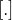$[.]$ represents the greatest integer function.
 Solution: 4
One might say that since the terms in the numerator are all integral, the numerator is not continuous and hence the limit will not exist. However note first of all that the limit is on n, and the secondly, addition of a large number of integral terms in the numerator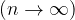$\left( {n \to \infty } \right)$ would tend to ‘overcome’ or ‘make negligible’ the effect of dfractional parts that would otherwise have been present had there been no greatest integer functions on any of the terms. This implies that whether I consider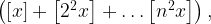$\left( {\left[ x \right] + \left[ {{2^2}x} \right] + \ldots\left[ {{n^2}x} \right]} \right),$ or ,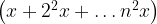$\left( {x + {2^2}x +\ldots {n^2}x} \right)$ as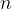$n$ becomes larger and , the difference between these two terms becomes negligible in comparison to their own magnitude. Hence, the limit in question is equivalent to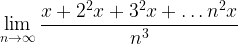$\mathop {\lim }\limits_{n \to \infty } \dfrac{{x + {2^2}x + {3^2}x +\ldots{n^2}x}}{{{n^3}}}$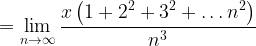$= \mathop {\lim }\limits_{n \to \infty } \dfrac{{x\left( {1 + {2^2} + {3^2} +\ldots{n^2}} \right)}}{{{n^3}}}$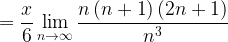$= \dfrac{x}{6}\mathop {\lim }\limits_{n \to \infty } \dfrac{{n\left( {n + 1} \right)\left( {2n + 1} \right)}}{{{n^3}}}$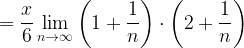$= \dfrac{x}{6}\mathop {\lim }\limits_{n \to \infty } \left( {1 + \dfrac{1}{n}} \right) \cdot \left( {2 + \dfrac{1}{n}} \right)$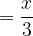$= \dfrac{x}{3}$
For students who like more rigor here is the proof of the above result using Sandwich theorem (In this proof, it will become clear that the effect of the dfractional part’s is negligible as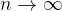$n \to \infty$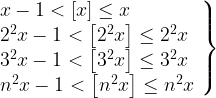$\left. \begin{array}{l} x - 1 < \left[ x \right] \le x\\ {2^2}x - 1 < \left[ {{2^2}x} \right] \le {2^2}x\\ {3^2}x - 1 < \left[ {{3^2}x} \right] \le {3^2}x\\ {n^2}x - 1 < \left[ {{n^2}x} \right] \le {n^2}x \end{array} \right\}$ By definiation of the greatest interger function
Addition of the these inequalities yields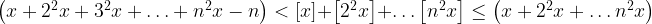$\left( {x + {2^2}x + {3^2}x +\ldots+ {n^2}x - n} \right) < \left[ x \right] + \left[ {{2^2}x} \right] +\ldots\left[ {{n^2}x} \right] \le \left( {x + {2^2}x +\ldots{n^2}x} \right)$
Division by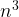${n^3}$ and application of on all three terms yields: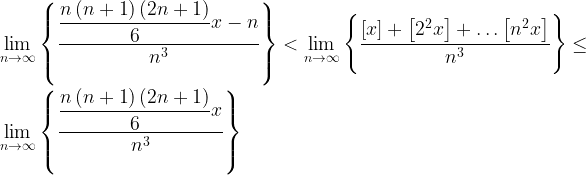$\mathop {\lim }\limits_{n \to \infty } \left\{ {\dfrac{{\dfrac{{n\left( {n + 1} \right)\left( {2n + 1} \right)}}{6}x - n}}{{{n^3}}}} \right\} < \mathop {\lim }\limits_{n \to \infty } \left\{ {\dfrac{{\left[ x \right] + \left[ {{2^2}x} \right] + \dots\left[ {{n^2}x} \right]}}{{{n^3}}}} \right\} \le \mathop {\lim }\limits_{n \to \infty } \left\{ {\dfrac{{\dfrac{{n\left( {n + 1} \right)\left( {2n + 1} \right)}}{6}x}}{{{n^3}}}} \right\}$
It is easy to that the left and right limits are both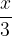$\dfrac{x}{3}$ , and hence the centre limit is also$\dfrac{x}{3}$.